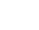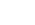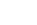# Physics: Electrostatics MCQs

## Getting Started with Physics: Electrostatics MCQ:

##### 1. Question

The flux linkage (λ) and current (i) relation for an electromagnetic system is = ( √ )/ . When i = 2A and (air-gap length) = 10 cm, the magnitude of mechanical force on the moving part, in N, is

`Answer`
##### 2. Question

A rotating conductor of 1 m length is placed in a radially outward (about the z-axis) magnetic flux  density (B) of 1 Tesla as shown in figure below. Conductor is parallel to and at 1 m distance from  the z-axis. The speed of the conductor in r.p.m. required to induce a voltage of 1 V across it, should be __________`Answer`
##### 3. Question

Consider a function f`= 1/r2 r̂  where r is the distance from the origin and r̂ is the unit vector in the radial direction. The divergence of this function over a sphere of radius R, which includes the origin, is

`Answer`
##### 4. Question

Consider a one-turn rectangular loop of wire placed in a uniform magnetic field as shown in the figure. The plane of the loop is perpendicular to the field lines. The resistance of the loop is 0.4 Ω, and its inductance is negligible. The magnetic flux density (in Tesla) is a function of time, and is given by B(t) = 0.25 sinωt, where ω = 2π × 50 radian/second. The power absorbed (in Watt) by the loop from the magnetic field is _________.`Answer`
##### 5. Question

A steady current I is flowing in the –x direction through each of two infinitely long wires at y = ± L/2 as shown in the figure. The permeability of the medium is μ0 . The B – field at (0, L, 0) is`Answer`